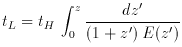### 10. LOOKBACK TIME

The lookback time tL to an object is the difference between the age t0 of the Universe now (at observation) and the age te of the Universe at the time the photons were emitted (according to the object). It is used to predict properties of high-redshift objects with evolutionary models, such as passive stellar evolution for galaxies. Recall that E (z) is the time derivative of the logarithm of the scale factor a (t); the scale factor is proportional to (1 + z), so the product (1 + z) E (z) is proportional to the derivative of z with respect to the lookback time, or(29)

(Peebles 1993, pp. 313-315; Kolb & Turner 1990, pp. 52-56, give some analytic solutions to this equation, but they are concerned with the age t (z), so they integrate from z to). The lookback time is plotted in Figure 6.Figure 6. The dimensionless lookback time tL / tH and age t / tH. The three curves are for the three world models, (M,) = (1, 0), solid; (0.05, 0), dotted; and (0.2, 0.8), dashed.